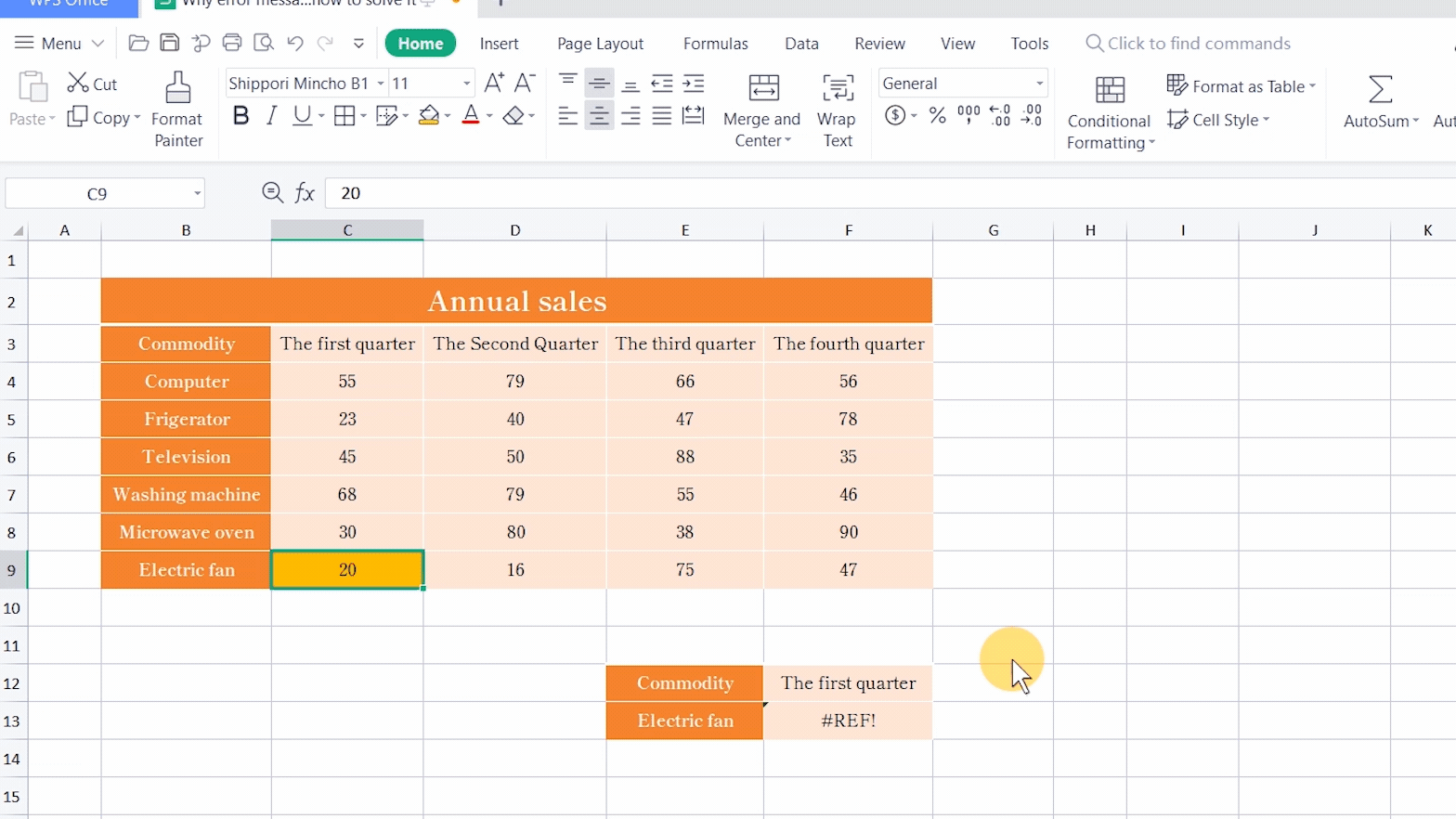# Why error message #REF! occurs and how to solve it

Uploaded time: March 2, 2022 Difficulty: Intermediate# Why error message #REF! occurs and how to solve it

The error message #REF is common in table calculations.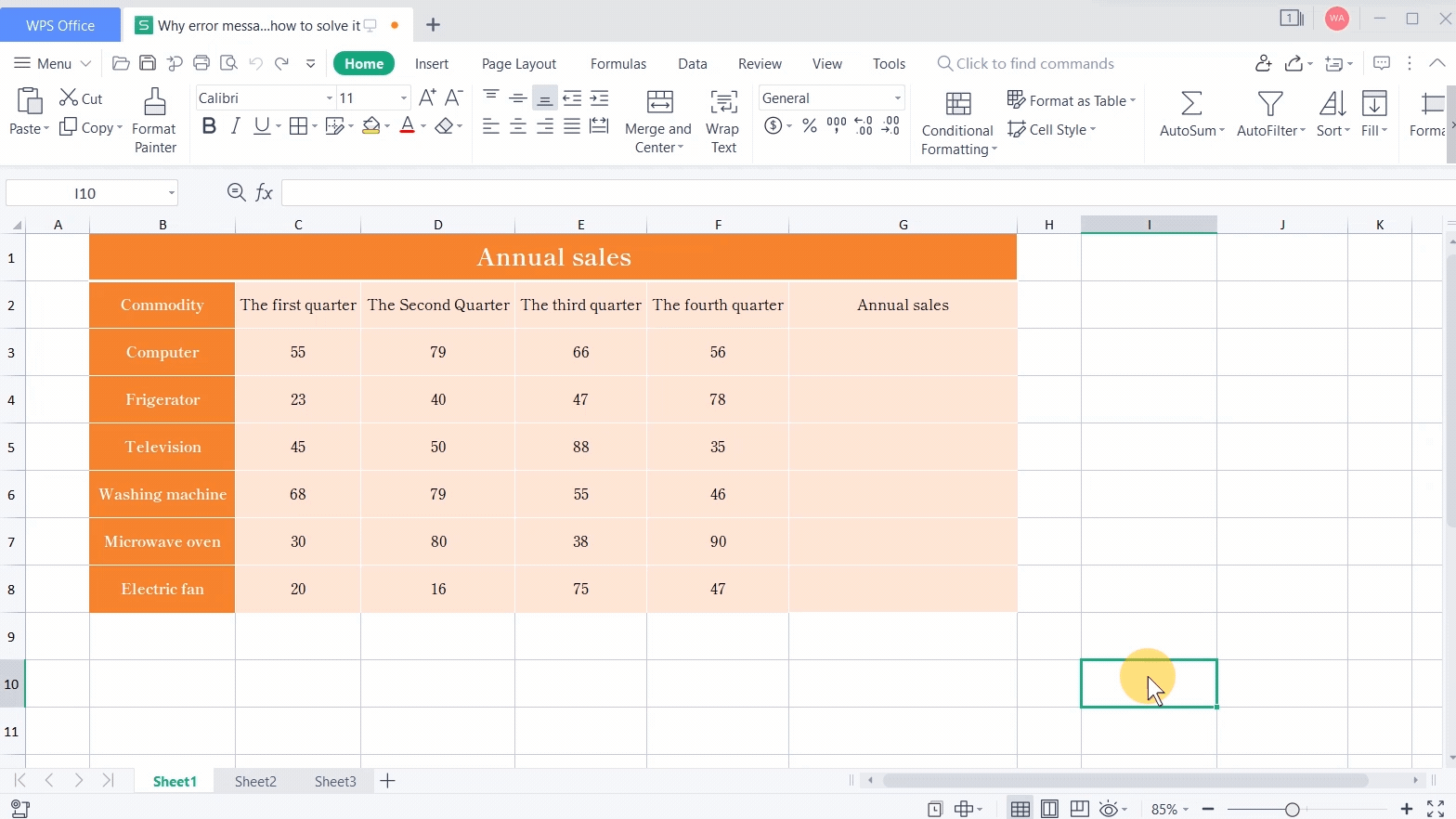In general, there are mainly two causes. First, the referenced cells in the formula are deleted or replaced by other cells. Second, the formula references invalid cell ranges or values.

Then how can we solve these problems?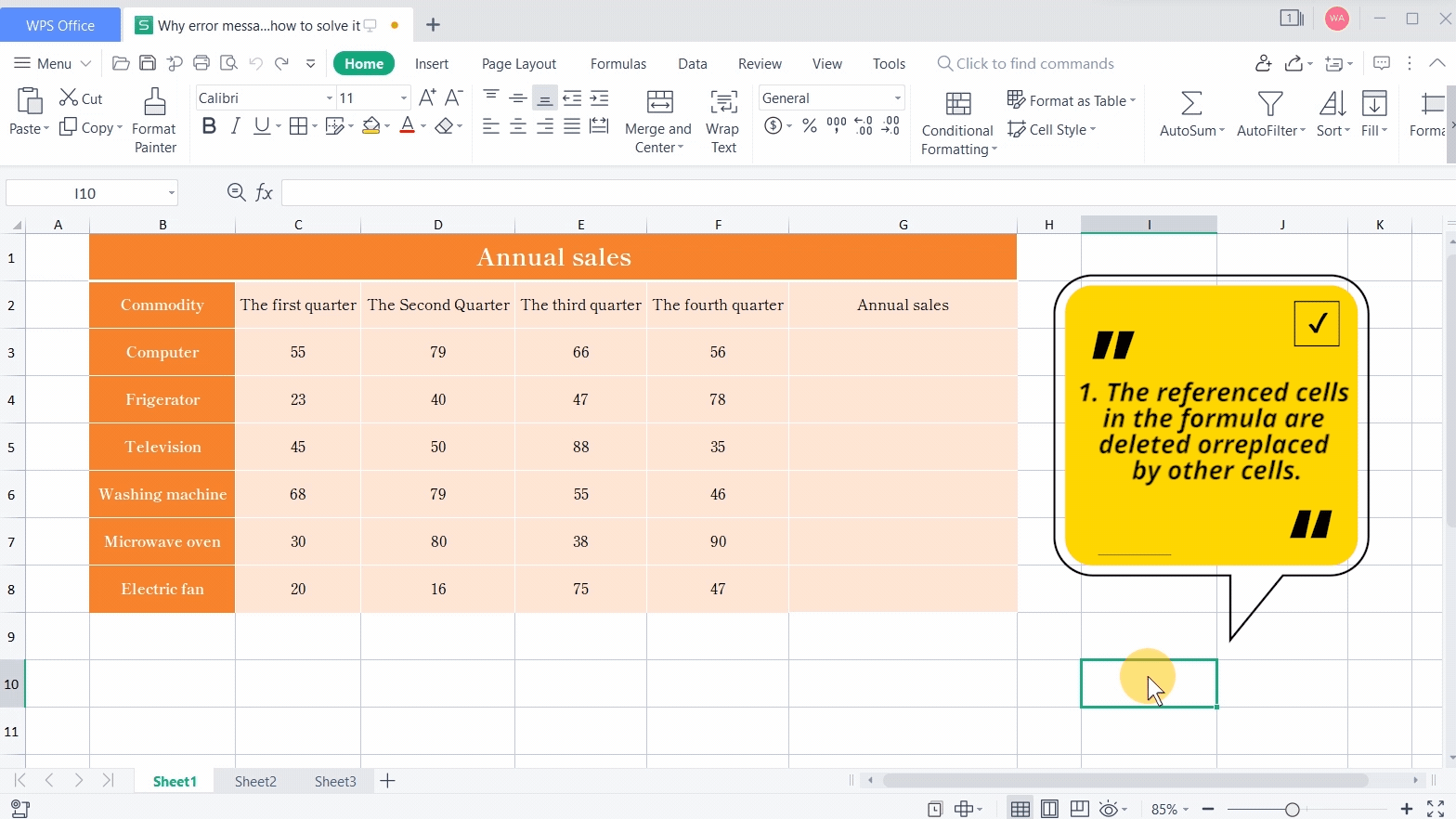· The referenced cells in the formula are deleted or replaced by other cells.

The referenced cells in the formula are deleted.

Take this table as an example.

1. To calculate the total sales of computers this year, input the formula =C3+D3+E3+F3 and press the Enter key to get the result.

2. If we delete column E, it returns #REF!.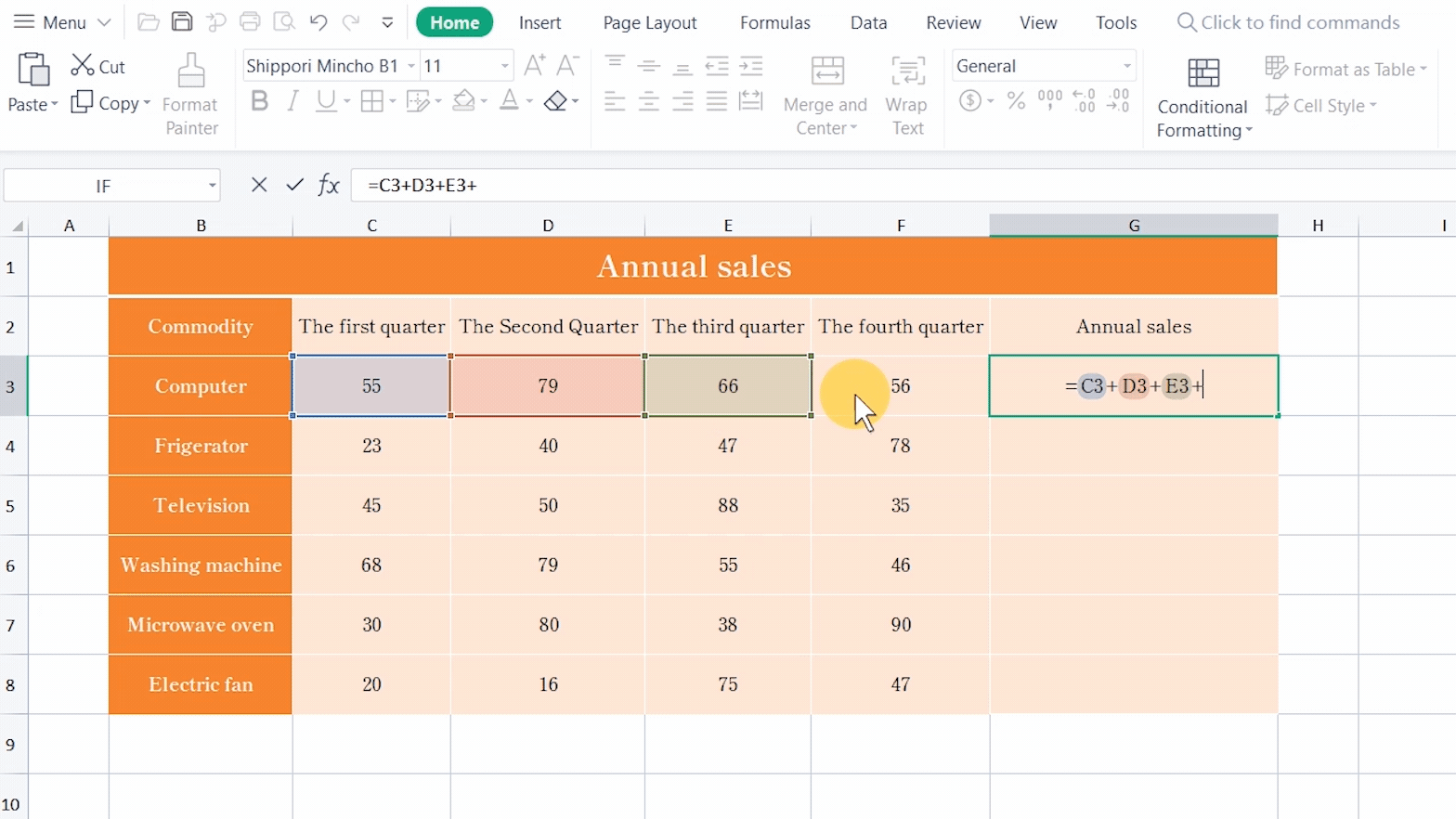That is due to the calculation error caused by the invalid cells because the referenced data in the formula is deleted.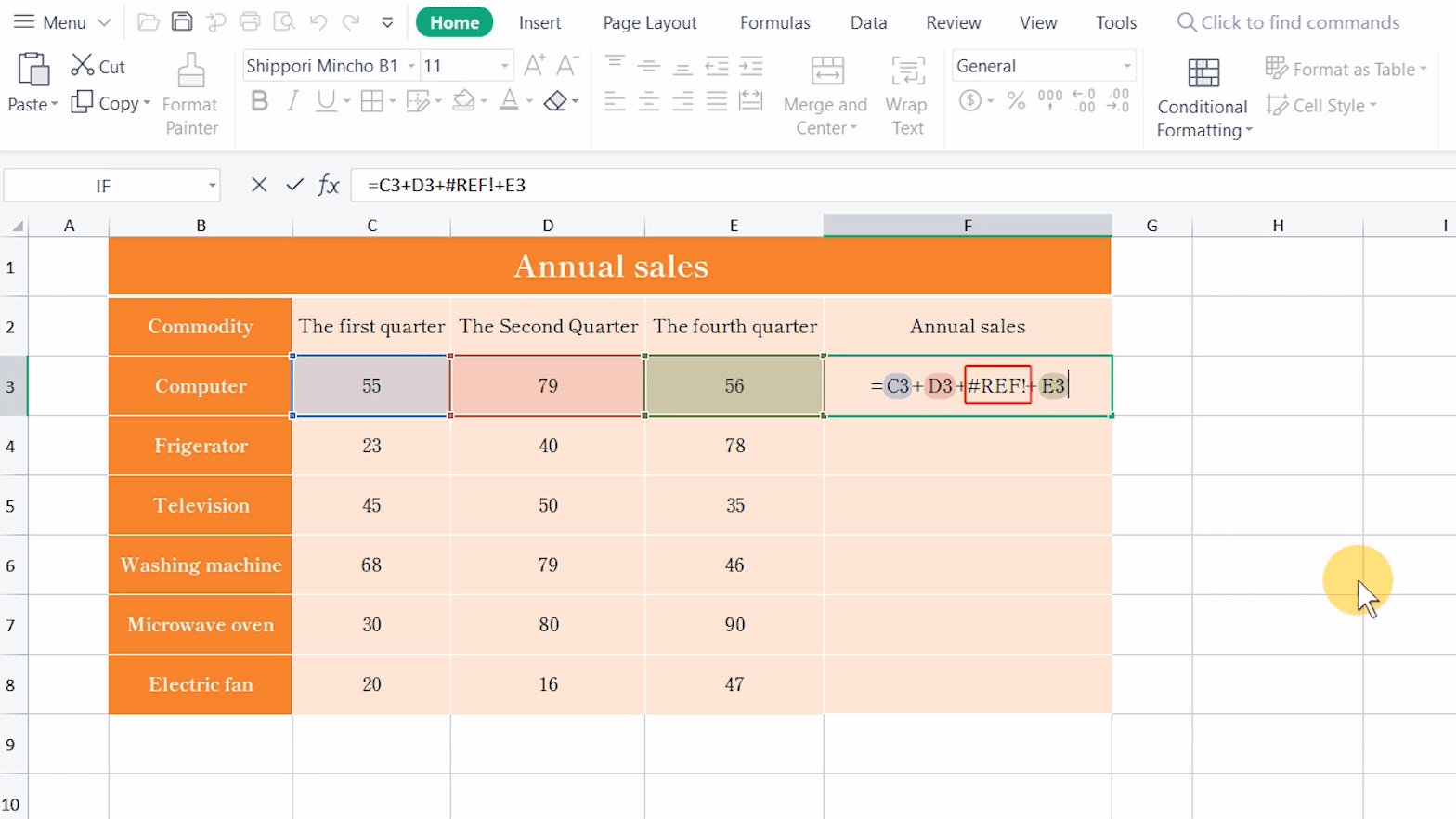The solution is to press the shortcut key Ctrl+Z to undo the previous step or fill in the data in the deleted column to return the correct results.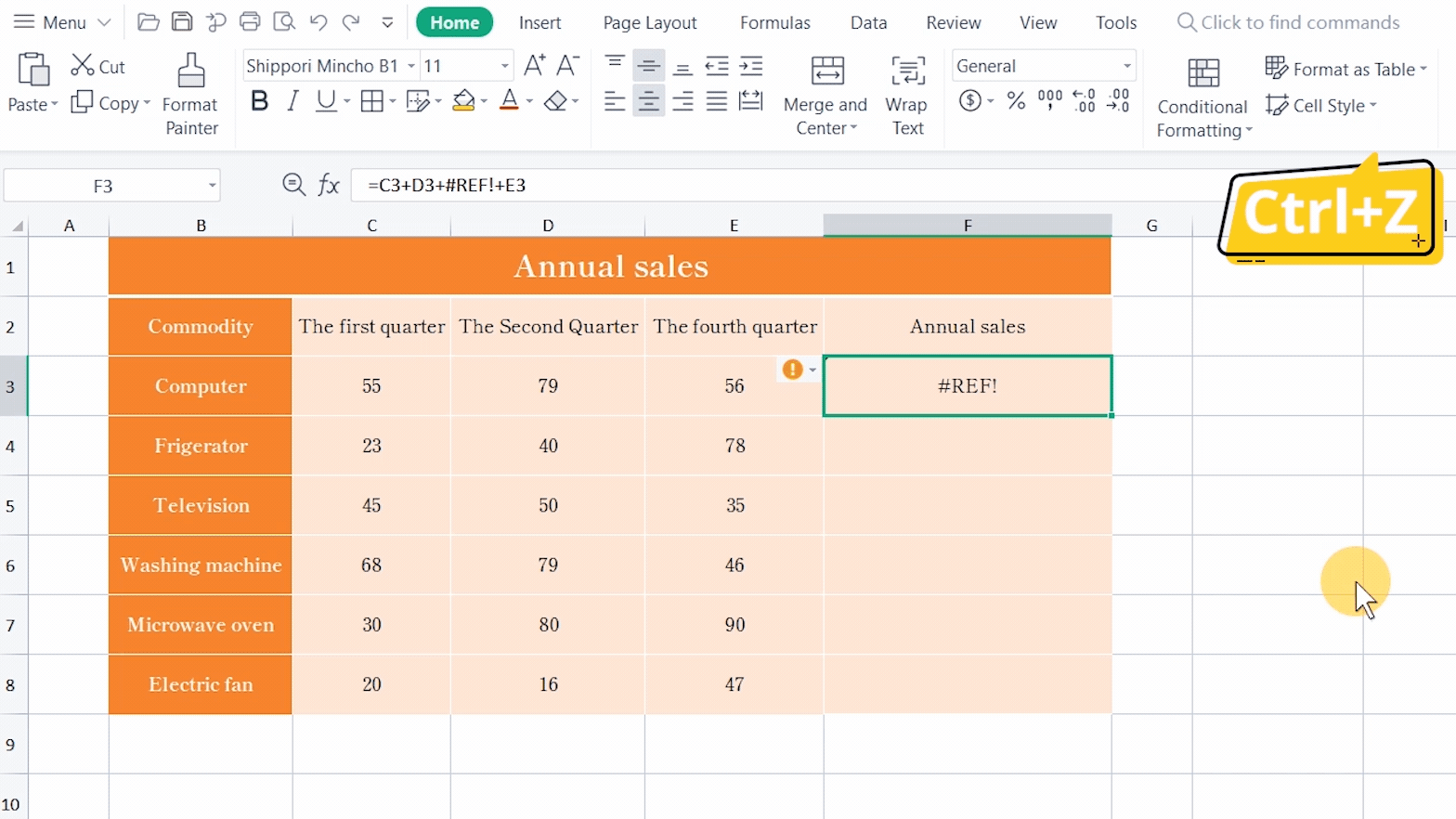The referenced cells in the formula are replaced by other cells.

We often need to replace the data in a table when making statements. Take this table as an example, which displays the annual sales of refrigerators.

1. Input the formula =SUM(B3:E3) to sum up the sales of four quarters and get the total sales of this year.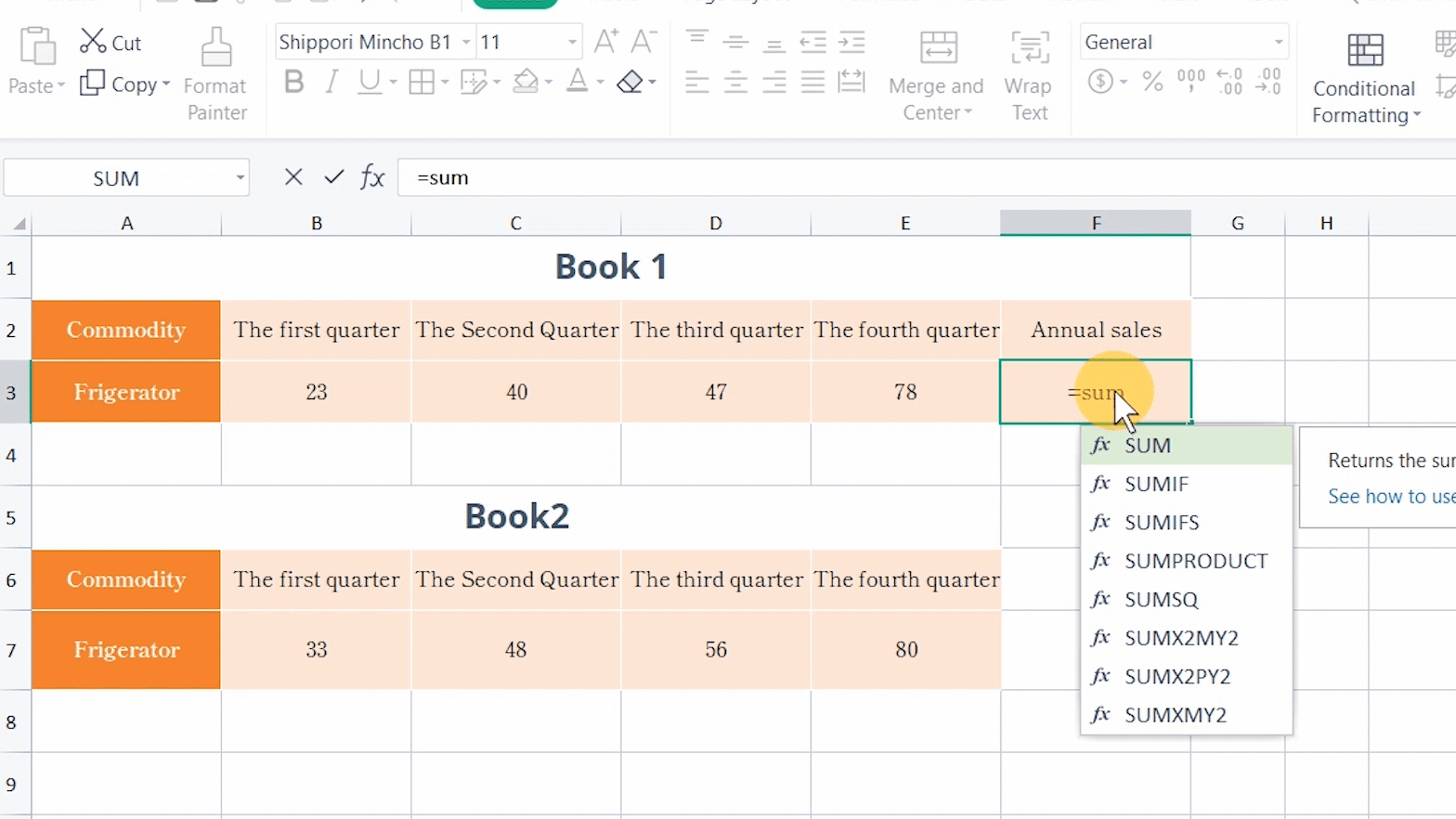2. Now we want to replace the data of Book 1 with that of Book 2 by dragging Book 2 directly.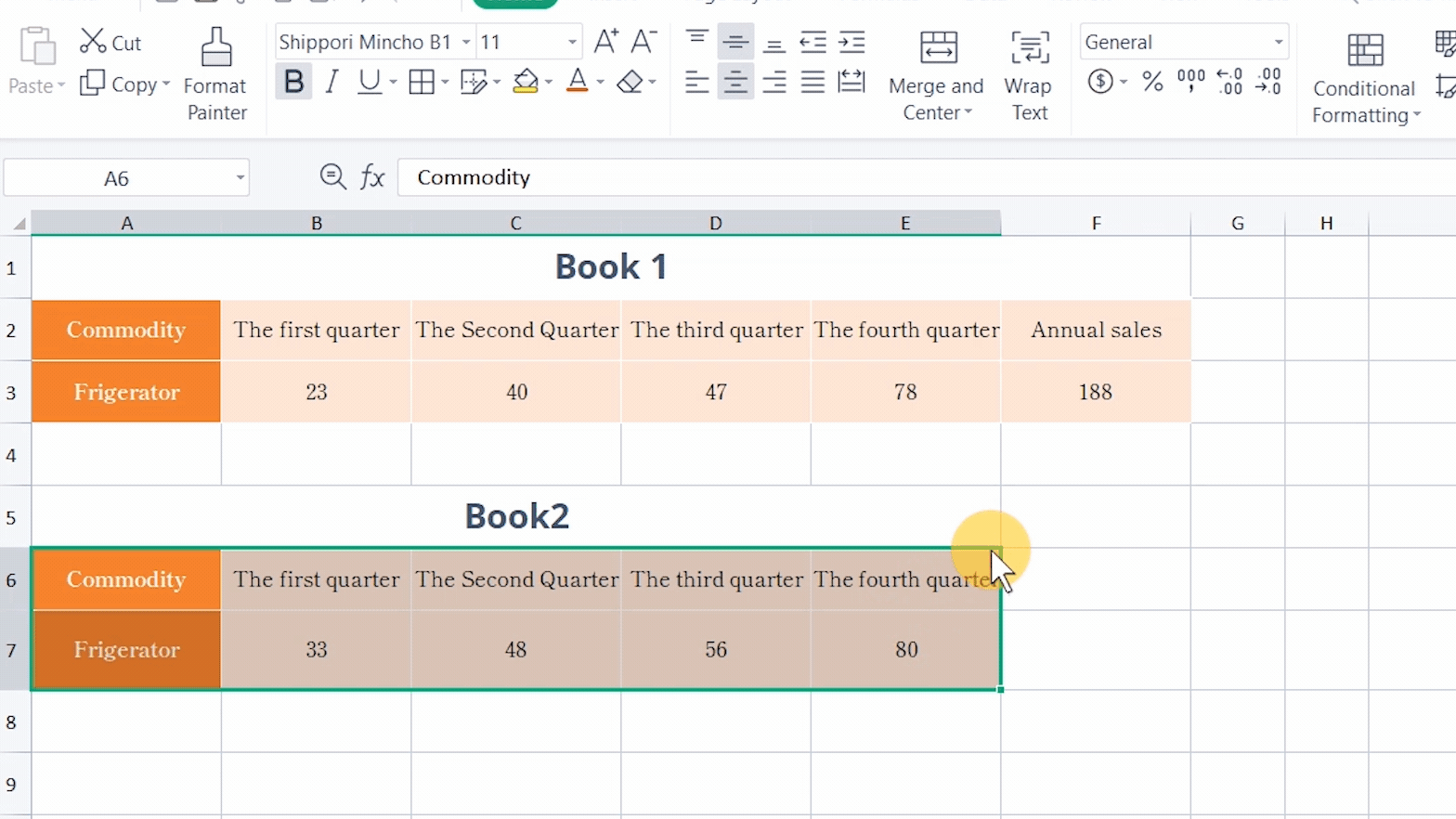3. Then it returns #REF! in  cell F3.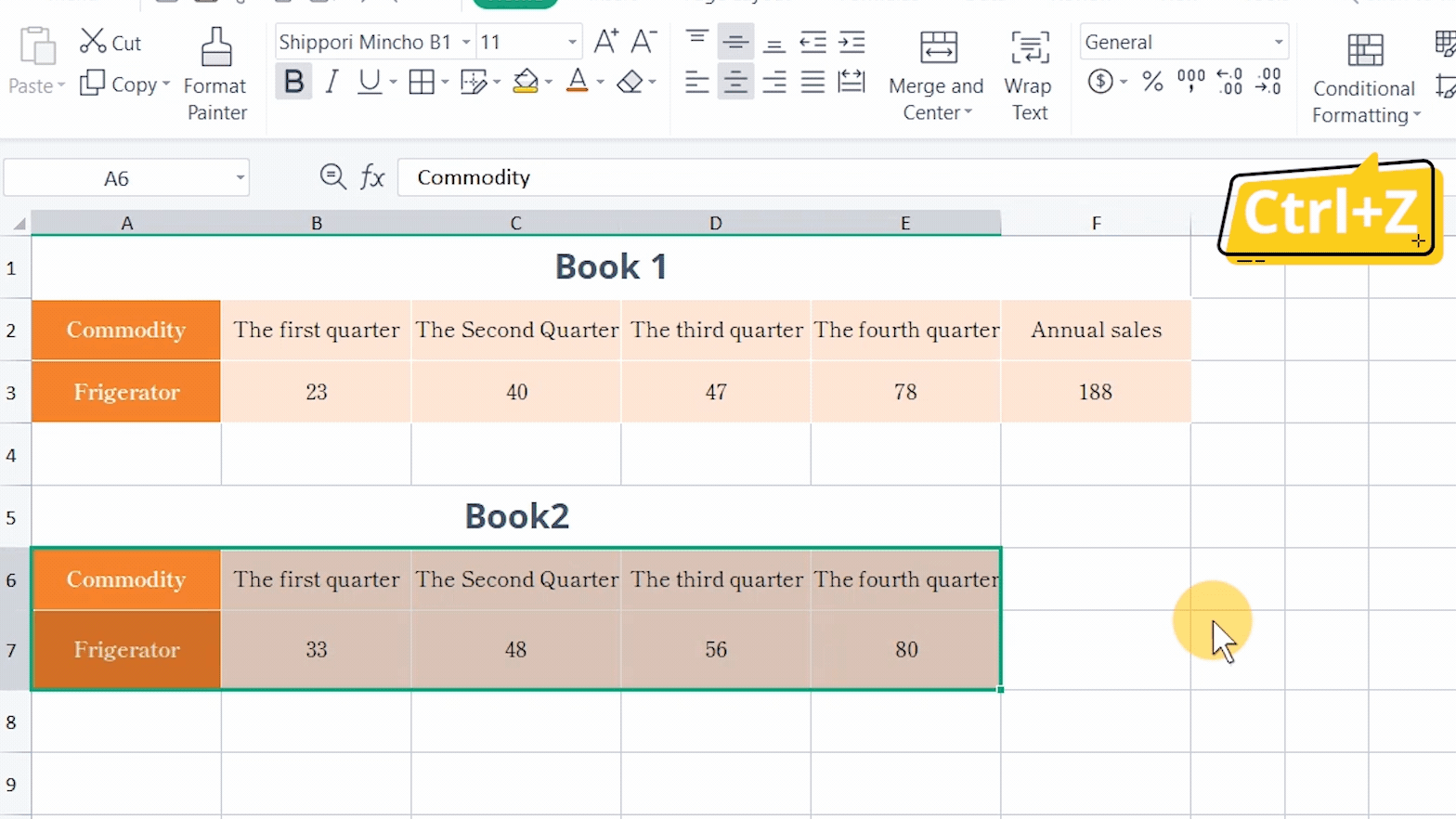That is due to the calculation error caused by the invalid cells because the referenced data in the formula are replaced by other cells.

The solution is to press the shortcut key Ctrl+Z to undo the previous step and press Ctr+C and Ctrl+V to update Book 1.· The formula references invalid cell ranges or values.

The INDEX function can be used to locate the position of a table according to the row and column.

If we want to check the sales of electric fans in the first quarter and we know the data is located in Row 9, Column 3, we can use the INDEX function. Input the formula =INDEX(B2:F9,9,3), but it returns #REF!.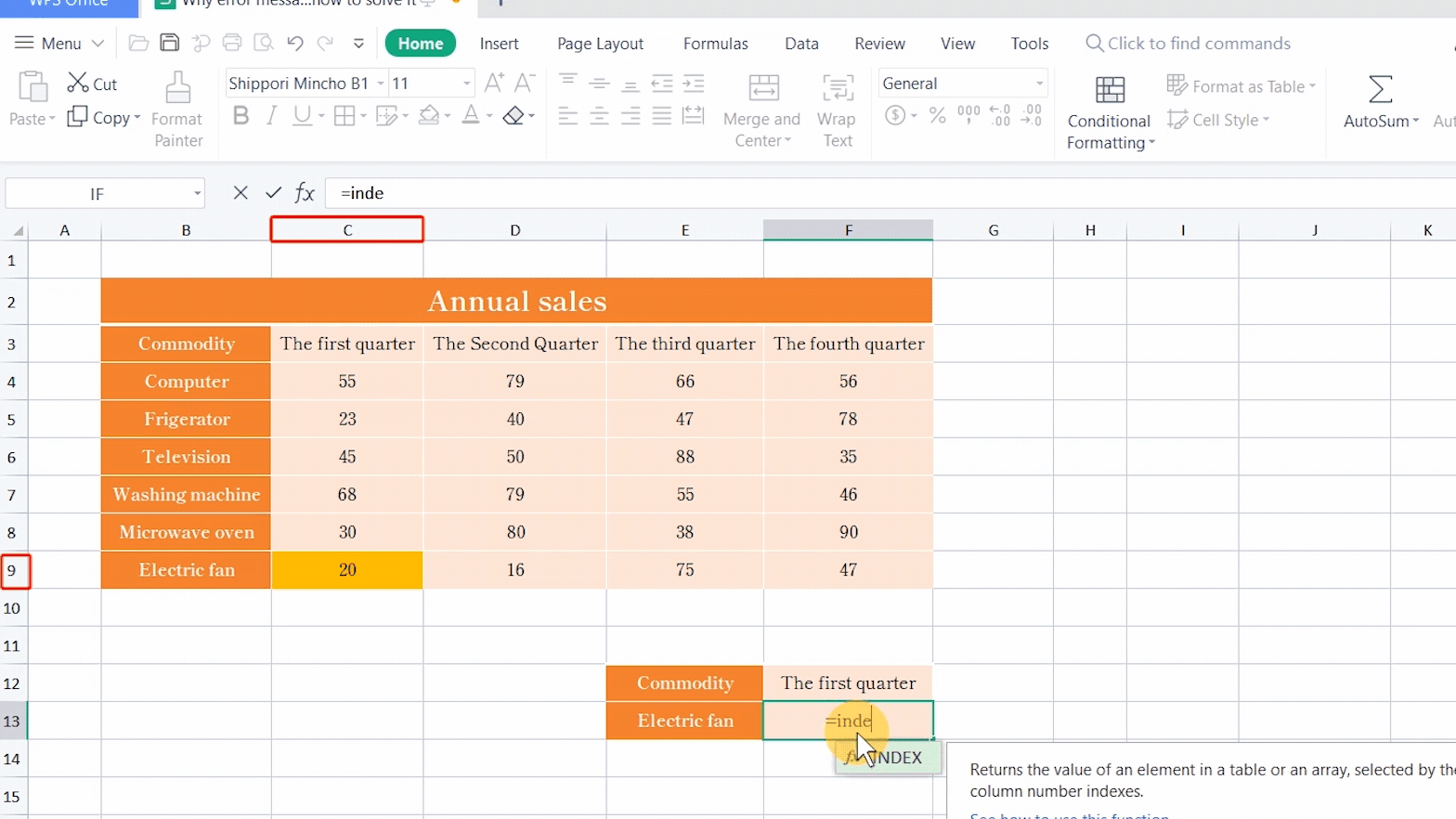There are two causes.

1. All functions can identify the selected cell range intelligently, and there are only eight rows in this table, but we input 9 as the row ordinal number.2. The position of the targeted cell is not based on the row and column of this table but the selected range.

Beginners to excel tend to make similar mistakes.

The solution is to correct the reference parameters. We can see that the selected cell range has eight rows and five columns, and the targeted cell is located in Row 8, Column 2. So we need to change the row ordinal number to 8 and the column ordinal number to 2.